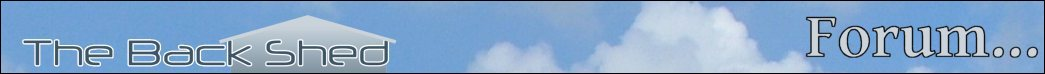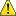Notice. New forum software under development. It's going to miss a few functions and look a bit ugly for a while, but I'm working on it full time now as the old forum was too unstable. Couple days, all good. If you notice any issues, please contact me.

Forum Index : Microcontroller and PC projects : CMM2 demo programs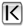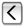Page 4 of 4
Author Message
TassyJim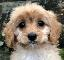Guru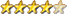Joined: 07/08/2011
Location: Australia
Posts: 3712
 Posted: 04:38am 28 Jul 2020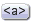Copy link to clipboard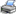Print this post

This is one I converted a while ago.

Comparing speeds with my PC,
computing Pi to 500 places,
on the PC
Total computation time:   94 mS
Time to format and print: 31 mS at 14:30:49

on the CMM2
Total computation time:   611 mS
Time to format and print: 17 mS at 14:30:27

slower to think but faster to talk...

' ported by TassyJim from a post on PureBasic forum  by Jeff88
'
';Many years ago when I used Quickbasic, I came across a program to calc pi.  Of course this has no practical
';purpose, but just to fight winter boredom I modified the program (see comments included below) to run
';under Purebasic,  now can use 10 digits per word instead of 4. Also added a bit of assembly to avoid calculating
';the remainder, this only makes a slight difference since most of time is taken by dividing.
';Using threads sped up by a factor of 3.  Note calc atan(1/5) takes about twice as long as atan(1/239).
';Programmed by Jeff Wyatt, Highlands Ranch, Colorado (1/2019).
'
';On my Athelon II X4 635  the program can calculate 100,000 digits in about 8 secs with debugging off.
'
';   >>>>>>>>>>>>>>>>>>>>>>>COMPILE With THREAD SAFE BOX CHECKED<<<<<<<<<<<<<<<<<<<<<<<<<<<<
'
';Based upon Machin's (1706) formula: pi/4=arctan(1)=4*arctan(1/5)-arctan(1/239), he did 100 digits by hand
';which would take about 8 usec with this program.  Time is prop. to digits^2 so need to use another method
';for very large number of digits. Good way to test your computer's integer arithmatic.
';See https://www.angio.net/pi/ for further info and searchable listing of pi.
';I used it to check that my program worked correctly for the first 2,000,000 digits.
'
';Previous comments from authors 20 years ago
'
';Program To calculate pi, version 4.8
';A major rewrite of version 4.2, this uses only two arrays instead of
';three, and includes a host of speedups based on a similar C program.
';A sampler: all the carries are reserved until the end, the divide and
';add routines are combined, two terms are added at a time, and the number
';of function calls is minimized. It's a big change for a small gain, since
';the compiled version requires 28.6 seconds for 5000 digits on my 486 66 MHz
';computer, a 10 gain over version 4.2; like before, it's capable of about
';150,000 digits of pi.
';
';This program has come a long way from version 1.0; thanks are due to
';Larry Shultis, Randall Williams, Bob Farrington and Adrian Umpleby.
';One final note for speed freaks: this program will run about 6 times faster
';if written in C using an optimizing compiler. Likewise, if you can figure
';out a way to do integer division and use both the quotient and remainder,
';this program can easily be sped up by 25.      -jasonp@isr.umd.edu
OPTION EXPLICIT
DIM INTEGER words, big, Digits, DigitsperWord, x, i, quotient
DIM INTEGER ctime, stime

DigitsperWord=10
big=1
FOR i=1 TO DigitsperWord  '1 followed by 10 zeros, note if you use 14 digits/word
big=big*10              'arctan(1/5) will overflow about digit 64,760
NEXT                      'when 1.4*digits*big~denom*big >9e18

INPUT "how many digits? ",Digits
words = Digits / DigitsperWord+2
DIM INTEGER sum(words + 2),sum1(words+2),sum2(words+2)
stime = TIMER
print "Started at "+time\$
'---------------  -4*atan(1/239)
x = atan239()
'------------   16*atan(1/5)
PRINT STR\$(TIMER - stime)+"mS so far"
x = atan51()
PRINT STR\$(TIMER - stime)+"mS so far"
x = atan52()
PRINT STR\$(TIMER - stime)+"mS so far"
sum1(2)=sum1(2)-big/5
FOR x=2 TO words
sum(x)=sum(x)+sum1(x)+sum2(x)
NEXT

FOR x = words TO 2 STEP -1                        'finish up
IF sum(x) < 0   THEN                                'release borrows
quotient = sum(x) \ big
sum(x) = sum(x) - (quotient - 1) * big
sum(x - 1) = sum(x - 1) + quotient - 1
ENDIF
IF sum(x) >= big  THEN                              'and carries
quotient = sum(x) \ big
sum(x) = sum(x) - quotient * big
sum(x - 1) = sum(x - 1) + quotient
ENDIF
NEXT
ctime = TIMER - stime
x = PrintOut()

END

FUNCTION atan239()                    'arctan(x) = x-x^3/3+x^5/5-x^7 ....
LOCAL INTEGER mainder, mainder1, mainder2, dividend, denom, temp, firstword, x
LOCAL INTEGER term(words +2), lastword
mainder=4
FOR x = 2 TO words
dividend = mainder * big              'crunch out 1st term
term(x) = dividend \ 239
mainder = dividend - term(x) * 239
sum2(x) = sum2(x) - term(x)             'subtract as we want -atan(1/239)
NEXT x

denom=3:firstword=2
DO                                   'do two more terms, add first, subtract second
mainder1=0
mainder2=0
FOR x = firstword TO words
temp = term(x)
dividend = mainder1 * big + temp
temp = dividend \ 57121
mainder1 = dividend - temp * 57121
term(x) = temp
dividend = mainder2 * big + temp
temp = dividend \ denom
mainder2 = dividend - temp * denom
sum2(x) = sum2(x) + temp
NEXT

IF term(firstword) = 0 THEN
firstword = firstword + 1
ENDIF
denom = denom + 2
mainder1 = 0: mainder2 = 0

FOR x = firstword TO words
temp = term(x)
dividend = mainder1 * big + temp
temp = dividend \ 57121
mainder1 = dividend - temp * 57121
term(x) = temp

dividend = mainder2 * big + temp
temp = dividend \ denom
mainder2 = dividend - temp * denom
sum2(x) = sum2(x) - temp
NEXT x

IF term(firstword) = 0 THEN
firstword = firstword + 1
ENDIF
denom = denom + 2
LOOP UNTIL firstword >=words
END FUNCTION
'-------------------------------------------------------------------
FUNCTION atan51()                      ' atan(1/5)  one half of calc terms 1/5, 1/9, 1/13 ......
LOCAL INTEGER mainder, mainder1, mainder2, dividend, denom, temp, firstword, x
LOCAL INTEGER term(words +2), lastword
denom = 5: firstword = 1: lastword =3
sum(1) = 3: term(1) = 3: sum(2) = big/5: term(2) = sum(2)
DO

mainder1=0
mainder2=0
FOR x = firstword TO lastword + 1
temp = term(x)
dividend = mainder1 * big + temp
temp = dividend \ 625                 ' 625 = 5^4
mainder1 = dividend - temp * 625
term(x) = temp

dividend = mainder2 * big + temp
temp = dividend \ denom
mainder2 = dividend - temp * denom
sum(x) = sum(x) + temp
NEXT

FOR x = lastword + 2 TO words
dividend = mainder2 * big
temp = dividend \ denom
mainder2 = dividend - temp * denom
sum(x) = sum(x) + temp
NEXT
IF term(lastword + 1) > 0 AND lastword < words THEN
lastword = lastword + 1
ENDIF
IF term(firstword) = 0 THEN
firstword = firstword + 1
ENDIF

denom = denom + 4
LOOP UNTIL firstword >= words

END FUNCTION

FUNCTION atan52()             ' atan(1/5)  other half of calc terms 1/3, 1/7, 1/11 ....
LOCAL INTEGER mainder, mainder1, mainder2, dividend, denom, temp, firstword, x
LOCAL INTEGER term(words +2), lastword
denom = 3: firstword = 1: lastword =3
sum1(1) = 3: term(1) = 3: sum1(2) = big/5: term(2) = sum1(2)
print "starting atan52()" 'DEBUG
mainder1=0
mainder2=0
FOR x = firstword TO lastword + 1   'term 1/3
temp = term(x)
dividend = mainder1 * big + temp
temp = dividend \ 25
mainder1 = dividend - temp * 25
term(x) = temp

dividend = mainder2 * big + temp
temp = dividend \ denom
mainder2 = dividend - temp * denom
sum1(x) = sum1(x) - temp
NEXT
print "atan52() step 2" 'DEBUG
FOR x = lastword + 2 TO words
dividend = mainder2 * big
temp = dividend \ denom
mainder2 = dividend - temp * denom
sum1(x) = sum1(x) - temp
NEXT
IF term(lastword + 1) > 0 AND lastword < words THEN
lastword = lastword + 1
ENDIF
IF term(firstword) = 0 THEN
firstword = firstword + 1
ENDIF
denom = denom + 4
print "atan52() step 3" 'DEBUG
DO                                'do the rest
if firstword mod 100 = 0 then print firstword 'DEBUG
mainder1 = 0: mainder2 = 0
FOR x = firstword TO lastword + 1
temp = term(x)
dividend = mainder1 * big + temp
temp = dividend \ 625
mainder1 = dividend - temp * 625
term(x) = temp

dividend = mainder2 * big + temp     'eventually this will overflow >9e18
temp = dividend \ denom
mainder2 = dividend - temp * denom
sum1(x) = sum1(x) - temp
NEXT x

FOR x = lastword + 2 TO words
dividend = mainder2 * big
temp = dividend \ denom
mainder2 = dividend - temp * denom
sum1(x) = sum1(x) -temp
NEXT x

IF term(lastword + 1) > 0 AND lastword < words THEN
lastword = lastword + 1
ENDIF
IF term(firstword) = 0 THEN
firstword = firstword + 1
ENDIF
denom = denom + 4
LOOP UNTIL firstword >= words
END FUNCTION

FUNCTION PrintOut()
LOCAL p\$, i AS INTEGER, j AS INTEGER, ptime AS INTEGER
PRINT ""
p\$="pi = 3."
i=2
DO
FOR j=i TO i+4
IF j>words THEN
p\$=p\$+SPACE\$(11)
ELSE
p\$=p\$+STR\$(sum(j),10,0,"0")+" "
ENDIF
NEXT
p\$=p\$+STR\$(10*i+30,7)
PRINT p\$
p\$="       "
i=i+5
LOOP UNTIL i>=words
ptime = TIMER - stime - ctime
PRINT ""

PRINT " Total computation time:   "+STR\$(ctime)+" mS"
PRINT " Time to format and print: "+STR\$(ptime)+" mS at "+time\$
END FUNCTION

Jim
It all started with the ZX81....
VK7JH
http://www.c-com.com.au/MMedit.htm

goc30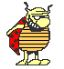GuruJoined: 12/04/2017
Location: France
Posts: 337
 Posted: 02:05am 31 Jul 2020Copy link to clipboardPrint this post

hi all

YESSS my CMM2 is working.

i have upgraded my graphical tests for CMM2

test all graphics functions
testgraf.zip

test fonts

test_fonts_cmm2.zip
Edited 2020-07-31 12:06 by goc30

capsikin
GuruJoined: 30/06/2020
Location: Australia
Posts: 332
 Posted: 04:45am 03 Aug 2020Copy link to clipboardPrint this post

MauroXavier said  New demoscene for Colour Maximite 2

Soon I will publish a site with all my source codes.

And you did. I think I found the demo code here: https://github.com/mauroxavierneto/psgmini_cmm2
(psgdemo.bas is the file to run)
Quote
I hope you enjoy the video!

It was great!

MauroXavier
Senior MemberJoined: 06/03/2016
Location: Brazil
Posts: 175
 Posted: 11:35am 03 Aug 2020Copy link to clipboardPrint this post

My GitHub repository doesn´t have half of my source codes because I need to fix some of them to not cause rage in the users because there are a LOT OF BUGS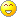I have a bad habit to make a lot of experiences thinking about my satisfaction, as programming on CMM2 is a hobby.

With a growing community, I must think about a better code indentation, comments and running with a minimum bug count, then now I guess it's better to fix my sources to a bare minimum quality before hosting them.

capsikin
GuruJoined: 30/06/2020
Location: Australia
Posts: 332
 Posted: 02:26pm 12 Aug 2020Copy link to clipboardPrint this post

abraxas said
thwill said  For kicks-and-giggles I am giving "Lunar" a kick to see if I can can persuade it to run on the CMM2. If anyone thinks I am treading on their toes and would rather I left it as an exercise for them then please let me know.

Tom

Not treading as I'm not doing working on that specific one but my hope is to eventually make a few 80's remakes for the CMM2 starting with a Boulder Dash clone followed by other 80's titles that I have a strong nostalgia for: Jet Pac, MinerWilly series, Montezuma's Revenge and many others. Someone's already done a very usable remake of ManicMiner from what I saw. I'll probably take on Montezuma's Revenge right after I'm done with my Boulder Dash clone.

I could only find Manic Miner for the old CMM, I don't think it's been ported to the CMM2.

Version I found:
https://www.thebackshed.com/forum/ViewTopic.php?TID=11691&PID=140143#140143

I also liked Jet Pac, and Joust (which reminded me of it because of gravity, flying, platforms, and single screen levels)

TassyJimGuruJoined: 07/08/2011
Location: Australia
Posts: 3712
 Posted: 03:29am 21 Aug 2020Copy link to clipboardPrint this post

I have updated the display test card program to cater for the ever increasing number of diplay modes.
I have changed things to make it easier to navigate (I hope)

UP/DOWN arrows to change display resolution
LEFT/RIGHT arrows to change colour depth
+- keys to change circle aspect ratio
P to save a BMP image of the screen.
Q to quit

' test card for CMM2
' TassyJim August 2020

OPTION EXPLICIT
OPTION DEFAULT NONE
DIM INTEGER wd, ht, wbox, sh, x, w, n, nn, m, cd, maxMode, keepMode
DIM FLOAT a, defaultMode
DIM k\$, imgtitle\$, fname\$, imgRes\$
DIM INTEGER c(8)
c(0) = RGB(BLACK)
c(1) = RGB(YELLOW)
c(2) = RGB(CYAN)
c(3) = RGB(GREEN)
c(4) = RGB(MAGENTA)
c(5) = RGB(RED)
c(6) = RGB(BLUE)
c(7) = RGB(WHITE)
c(8) = RGB(64,64,64)

maxMode = 9
cd = 8
a = 1
defaultMode = MM.INFO(MODE)
CLS

DO
IF m = 0 THEN
MODE 1,8
CLS
TEXT 400,100, "Video mode test",cm,5,1
TEXT 400,180, "Ratio = aspect ratio used in the circle command",cm,1,1
TEXT 400,220, "Q to quit, P to save page as a BMP",cm,3,1
TEXT 400,260, "Up Down arrow to change resolution",cm,3,1
TEXT 400,300, "Left Right arrow to change colour depth",cm,3,1
TEXT 400,340, "+ - to change circle aspect ratio",cm,3,1
ELSE
IF keepmode THEN ' only change resolution if needed
keepmode = 0
ELSE
MODE m,cd
ENDIF
wd = MM.HRES : ht = MM.VRES
nn = INT(wd/80)
imgtitle\$ =" MODE "+STR\$(MM.INFO(MODE))+" Ratio "+STR\$(a,1,3)+" "
imgRes\$ = " "+STR\$(MM.HRES)+" x "+STR\$(MM.VRES)+" "
wbox = wd / 8
PAGE WRITE 1 ' no flicker during write
CLS
' primary colours
FOR x = 0 TO 7
BOX x*wbox,ht/4,wbox,ht/2,0,c(x), c(x)
NEXT x
' full gradient for each primary colour and greyscale
FOR x = 0 TO wd-1
sh = 255*x/wd

LINE x,0,x,ht/12,1,RGB(sh,0,0)
LINE x,ht/12,x,ht/6,1,RGB(0,sh,0)
LINE x,ht/6,x,ht/4,1,RGB(0,0,sh)

LINE x,ht*9/12,x,ht*10/12,1,RGB(0,sh,sh)
LINE x,ht*10/12,x,ht*11/12,1,RGB(sh,0,sh)
LINE x,ht*11/12,x,ht,1,RGB(sh,sh,0)

LINE x,ht/2,x,ht*3/4,1,RGB(sh,sh,sh) ' greyscale
NEXT x
' circle to check aspect ratio
CIRCLE wd/2,ht/2, ht*15/32,3,a,c(7)
sh = 0
x = wd/2 - 55*nn/2
' black white bars to check monitor bandwidth
FOR w = 10 TO 1 STEP -1
FOR n = 1 TO nn
sh = 255 - sh
LINE x,ht*3/8,x,ht*5/8,w,RGB(sh,sh,sh)
x = x + w
NEXT n
NEXT w
' white and red border to check that image fits on monitor
BOX 0,0,wd,ht,3,c(7)
BOX 1,1,wd-2,ht-2,1,c(5)
' title
IF wd > 600 THEN
TEXT wd/2,ht/2, imgtitle\$,cm,4,1
TEXT wd/2,ht/2+25, imgRes\$,cm,4,1
ELSE
TEXT wd/2,ht/2, imgtitle\$,cm,1,1
TEXT wd/2,ht/2+25, imgRes\$,cm,1,1
ENDIF
' show the new image
PAGE COPY 1 TO 0 ,B
ENDIF
' wait for keypress
DO
k\$ = INKEY\$
LOOP UNTIL k\$<>""
'
SELECT CASE k\$
CASE "Q","q"
EXIT DO
CASE "P","p"
fname\$ = MID\$(imgtitle\$,2)+".bmp"
TIMER = 0
SAVE IMAGE fname\$
PAGE WRITE 0
TEXT wd/2,ht/2,"Saved as "+fname\$+" in "+STR\$(TIMER/1000,3,2)+" Sec" ,cm,1,1
DO
k\$ = INKEY\$
LOOP UNTIL k\$<>""
CASE CHR\$(128) ' up arrow
m = m - 1
IF m < 1 THEN m = maxMode
CASE CHR\$(129) ' down arrow
m = m + 1
IF m > maxMode THEN m = 1
CASE CHR\$(131) ' right arrow res up
cd = cd + 4
IF cd > 16 THEN cd = 8
IF m = 9 AND cd = 12 THEN cd = 16 ' skip 12 bit for mode 9
CASE CHR\$(130) ' left arrow  res down
cd = cd - 4
IF cd < 8 THEN cd = 16
IF m = 9 AND cd = 12 THEN cd = 8 ' skip 12 bit for mode 9
CASE "+" ' ratio plus
IF a < 1.4 THEN a = a + 0.01
keepmode = 1
CASE "-" ' ratio minus
IF a > 0.75 THEN a = a - 0.01
keepmode = 1
CASE ELSE ' same as down arrow
m = m + 1
IF m > maxMode THEN m = 1
END SELECT
LOOP
setmode defaultMode ' restore original mode before ending program
PAGE WRITE 0
CLS

END

SUB setmode dotMode AS FLOAT
LOCAL INTEGER mm, md
' use float returned by mm.info(mode) to set MODE
mm = INT(dotmode)
md = (dotmode - mm)*100
IF md > 20 THEN md = md/10
MODE mm, md
END SUB

Jim
It all started with the ZX81....
VK7JH
http://www.c-com.com.au/MMedit.htm

thwillGuruJoined: 16/09/2019
Location: United Kingdom
Posts: 711
 Posted: 09:14am 02 Sep 2020Copy link to clipboardPrint this post

Jim,

Do you think (or want) your test card program to be included on the "Welcome Tape" ?

Best wishes,

Tom
CMM2 Welcome Tape, Z-MIM: Zork on the CMM2

SasquatchSenior MemberJoined: 08/05/2020
Location: United States
Posts: 159
 Posted: 02:06pm 02 Sep 2020Copy link to clipboardPrint this post

Let me do some work on the Mandelbrot, I plan to make it interactive so you can pan and zoom using the Keyboard or Nunchuk.

What about VegiPete's excellent Falfus2 puzzle game?

https://www.thebackshed.com/forum/ViewTopic.php?TID=12248&PID=148788#148788
-Carl

TassyJimGuruJoined: 07/08/2011
Location: Australia
Posts: 3712
 Posted: 09:27pm 02 Sep 2020Copy link to clipboardPrint this post

thwill said  Jim,

Do you think (or want) your test card program to be included on the "Welcome Tape" ?

Best wishes,

Tom

OK by me.
But change the maxModes to 10 first.

Jim
It all started with the ZX81....
VK7JH
http://www.c-com.com.au/MMedit.htm

HellbentHorse
Regular Member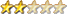Joined: 08/07/2020
Location: Australia
Posts: 51
 Posted: 08:42pm 05 Sep 2020Copy link to clipboardPrint this post

I am super new to the world of BASIC but had fun doing this on DOS MMBasic whilst I finish my CMM2 kit:

10 PRINT CHR\$(47+(INT(RND()*2)*45)); : GOTO 10

Hopefully one day I shall be at the same level as you MMBasic wizards.
Edited 2020-09-06 06:43 by HellbentHorse

mclout999
Senior MemberJoined: 05/07/2020
Location: United States
Posts: 109
 Posted: 09:37pm 06 Sep 2020Copy link to clipboardPrint this post

HellbentHorse said  I am super new to the world of BASIC but had fun doing this on DOS MMBasic whilst I finish my CMM2 kit:

10 PRINT CHR\$(47+(INT(RND()*2)*45)); : GOTO 10

Hopefully one day I shall be at the same level as you MMBasic wizards.

This is an adaptation of the 8bit Guys favorite 1 line programs of the C64, Nice.  I want to know if there are any fonts that have the \/ characters that go the whole-cell top to bottom like the C64's dose so there are not breaks in the sudo maze?  If not maybe I can make a custom font and load it.  I tried all the built in fonts on each of the 10 screens resolutions. Screen 1 and font 1 work the best so that is good but still a gape at every intersection.

vegipeteGuruJoined: 29/01/2013
Posts: 375
 Posted: 10:58pm 06 Sep 2020Copy link to clipboardPrint this post

Try this:
font 8

do : print CHR\$(48+(INT(RND*2))); : pause 25 : loop

DefineFont #8
03301010
02000100 08000400 20001000 80004000 00020001 00080004 00200010 00800040
00400080 00100020 00040008 00010002 40008000 10002000 04000800 01000200
00000000 00000000 00000000 00000000 00000000 00000000 00000000 00000000
End DefineFont

It's not a one liner anymore but it performs as required.

Adjust the pause as required. Without it, it's way too fast.

HellbentHorse
Regular MemberJoined: 08/07/2020
Location: Australia
Posts: 51
 Posted: 12:25am 07 Sep 2020Copy link to clipboardPrint this post

mclout999 said
This is an adaptation of the 8bit Guys favorite 1 line programs of the C64, Nice.  I want to know if there are any fonts that have the \/ characters that go the whole-cell top to bottom like the C64's dose so there are not breaks in the sudo maze?  If not maybe I can make a custom font and load it.  I tried all the built in fonts on each of the 10 screens resolutions. Screen 1 and font 1 work the best so that is good but still a gape at every intersection.

This was my only bug bear only using MMBasic on DOS at present, no support for custom fonts but it was on my to do list to revisit once I get to programming on my actual CMM2. Shouldn't be long now, just need to finish putting in the resistors and doing a final joints check.

TassyJimGuruJoined: 07/08/2011
Location: Australia
Posts: 3712
 Posted: 03:23am 07 Sep 2020Copy link to clipboardPrint this post

mclout999 said    I want to know if there are any fonts that have the \/ characters that go the whole-cell top to bottom like the C64's dose so there are not breaks in the sudo maze?  If not maybe I can make a custom font and load it.  I tried all the built in fonts on each of the 10 screens resolutions. Screen 1 and font 1 work the best so that is good but still a gape at every intersection.

The standard fonts deliberately have blank rows to have somewhere for the underline to go and make them readable.
If you want 'full' fonts, you will have to roll your own.

Jim
It all started with the ZX81....
VK7JH
http://www.c-com.com.au/MMedit.htm

mclout999
Senior MemberJoined: 05/07/2020
Location: United States
Posts: 109
 Posted: 03:44am 07 Sep 2020Copy link to clipboardPrint this post

TassyJim said
mclout999 said    I want to know if there are any fonts that have the \/ characters that go the whole-cell top to bottom like the C64's dose so there are not breaks in the sudo maze?  If not maybe I can make a custom font and load it.  I tried all the built in fonts on each of the 10 screens resolutions. Screen 1 and font 1 work the best so that is good but still a gape at every intersection.

The standard fonts deliberately have blank rows to have somewhere for the underline to go and make them readable.
If you want 'full' fonts, you will have to roll your own.

Jim

Good info, thanks.  That does make sense.  Now I get to figure out how to do custom fonts.  Would it be kosher to just copy, say font 1 and edit the two characters I want? If that is not cool then can you just make a 2 character font? I think I saw a program that had just a few characters in the define area.

EDIT:  I did not see the response above. People here are so fast to help and that is why this has been one of the best projects I have ever seen.  That and the amazing work by the developers that are shockingly fast at implementing tweaks and imaginative improvements.  Thanks all.
Edited 2020-09-07 13:48 by mclout999

mclout999
Senior MemberJoined: 05/07/2020
Location: United States
Posts: 109
 Posted: 03:52am 07 Sep 2020Copy link to clipboardPrint this post

vegipete said  Try this:
font 8

do : print CHR\$(48+(INT(RND*2))); : pause 25 : loop

DefineFont #8
03301010
02000100 08000400 20001000 80004000 00020001 00080004 00200010 00800040
00400080 00100020 00040008 00010002 40008000 10002000 04000800 01000200
00000000 00000000 00000000 00000000 00000000 00000000 00000000 00000000
End DefineFont

It's not a one liner anymore but it performs as required.

Adjust the pause as required. Without it, it's way too fast.

Nice, here I was thinking I would have to learn how to do this and Now I know how.  Awsome.  Thanks.  I do still need to figure out how defineFont works but now I have an example.  I tried it and it works in all modes.  Very nice.
Edited 2020-09-07 14:27 by mclout999

TassyJimGuruJoined: 07/08/2011
Location: Australia
Posts: 3712
 Posted: 10:08pm 19 Sep 2020Copy link to clipboardPrint this post

Updated test card program to include the new modes 10,11 and 12

colourBars.zip

edited with small update to show max page number in each mode

Jim
Edited 2020-09-20 10:39 by TassyJim
It all started with the ZX81....
VK7JH
http://www.c-com.com.au/MMedit.htmPage 4 of 4Print this page